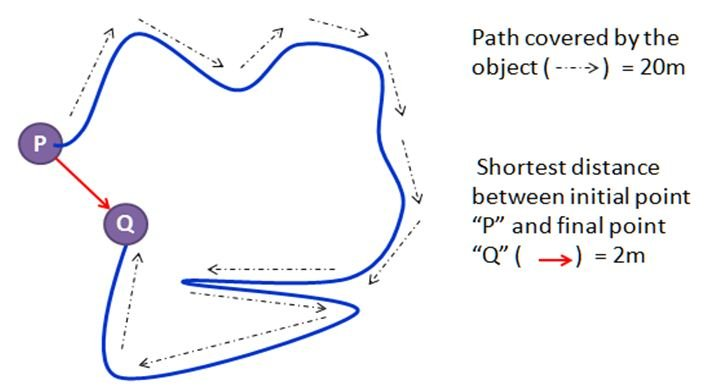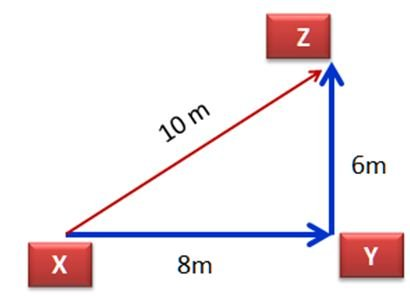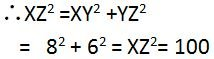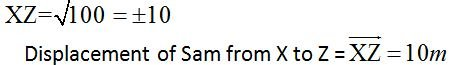# Definition Of Displacement

## Definition Of Displacement

The shortest distance between initial point and final point is known as Displacement.

The complete path covered by a body is not considered. Only the initial and final points are considered.
The "direction" is the deciding factor in displacement. Body/object must move in particular direction for determining the displacement.
Without considering the direction of body (through which it moved) it is not possible to determine the displacement.

Example: An object moved from P to Q in the following path, covering a distance of 20m. The distance between Initial point "P" and Final point "Q" is only 2m.The displacement of the object is only 2m.Displacement is measured in meters. Represented with an arrow on the top ‘ →’
In order to represent displacement magnitude and direction both are required .
In the above example: 2m is the magnitude and is the direction. (Object moved from P to Q).

#### Worked Example:

Sam starts from point ‘X’ and moved towards east for 8m reaches the point "Y" and turns to his left covers a distance of 6m to reach the point ‘Z’. Find the displacement of Sam from "X" to "Z"

A. 14m
B. 2m
C. 10m
D. 20m

#### SolutionStep 1: Draw a rough figure with the given measurements.
Step 2: The figure resembles a right triangle.
Step 3: Apply the ‘Pythagoras’ theory to find ‘XZ’.
Square of the hypotenuse is equal to sum of the squares of other two sides.
Step 4:Step 5:Related terms: Displacement | Speed, | Length.